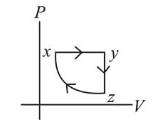# A thermodynamic cycle x y z x is shown on a V-T diagram.Question:

A thermodynamic cycle $x y z x$ is shown on a $V-T$ diagram.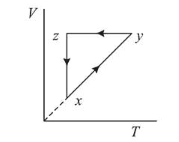The $P-V$ diagram that best describes this cycle is: (Diagrams are schematic and not to scale)

1.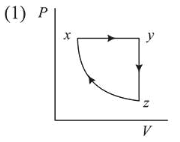2.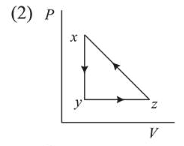3.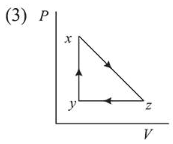4.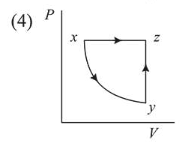Correct Option: 1

Solution:

(1) From the corresponding V-T graph given in question,

Process $x y \rightarrow$ Isobaric expansion,

Process $y z \rightarrow$ Isochoric (Pressure decreases)

Process $z x \rightarrow$ Isothermal compression

Therefore, corresponding $P V$ graph is as shown in

figure